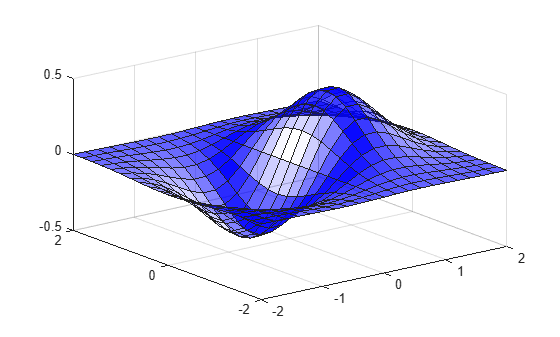alphamap

Specify figure alphamap (transparency)

Syntax

alphamap(alpha_map)
alphamap('parameter')
alphamap('parameter',length)
alphamap('parameter',delta)
alphamap(fig,...)
alphamap(ax,...)
alpha_map = alphamap
alpha_map = alphamap(fig)
alpha_map = alphamap(ax)
alpha_map = alphamap('parameter')

Description

alphamap(alpha_map) sets the AlphaMap of the current figure to the specified m-by-1 array of alpha values, alpha_map. If you set the alphamap for the figure, then axes and charts in the figure use the same alphamap.

alphamap('parameter') creates a new alphamap or modifies the current alphamap. You can specify the following parameters:

• 'default' — Set the AlphaMap property to the figure's default alphamap.

• 'rampup' — Create a linear alphamap with increasing opacity (default length equals the current alphamap length).

• 'rampdown' — Create a linear alphamap with decreasing opacity (default length equals the current alphamap length).

• 'vup' — Create an alphamap that is opaque in the center and becomes more transparent linearly towards the beginning and end (default length equals the current alphamap length).

• 'vdown' — Create an alphamap that is transparent in the center and becomes more opaque linearly towards the beginning and end (default length equals the current alphamap length).

• 'increase' — Modify the alphamap making it more opaque (default delta is .1, added to the current values).

• 'decrease' — Modify the alphamap making it more transparent (default delta is .1, subtracted from the current values).

• 'spin' — Rotate the current alphamap (default delta is 1; delta must be an integer).

alphamap('parameter',length) creates a new alphamap with the length specified by the integer length (used with parameters 'rampup', 'rampdown', 'vup', 'vdown').

alphamap('parameter',delta) modifies the existing alphamap using the value specified by the integer delta (used with parameters 'increase', 'decrease', 'spin').

alphamap(fig,...) performs the operation on the alphamap of the figure identified by fig.

alphamap(ax,...) performs the operation on the alphamap of the Axes object, PolarAxes object, or GeographicAxes object identified by ax.

alpha_map = alphamap returns the current alphamap.

alpha_map = alphamap(fig) returns the current alphamap from the figure identified by fig.

alpha_map = alphamap(ax) returns the current alphamap from the Axes object, PolarAxes object, or GeographicAxes object identified by ax.

alpha_map = alphamap('parameter') returns the alphamap modified by the parameter, but does not set the AlphaMap property.

Examples

collapse all

Create a surface plot and change the alphamap.

[x,y] = meshgrid([-2:.2:2]);
z = x.*exp(-x.^2-y.^2);

Plot the data, using the gradient of z as the alphamap.

figure
surf(x,y,z+.001,'FaceAlpha','flat',...
'FaceColor','blue')Change the alphamap to be opaque at the middle and transparent towards the ends.

alphamap('vup')